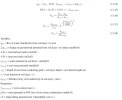# calculation of soil to root conductance

#### Crystal

##### New Member
To whom it may concern:

Hope everything goes well.
I'm writing to ask about the function of soil-to-root conductance in the plant hydraulic pathway.

In Chapter 11 of CLM5 tech note (page 120), the calculation of soil-to-root conductance is calculated by the eq. 11.16-11.18 and the water flux from soil to root is calculated by eq.11.14 (attached f1.jpg)However, when I checked the code about the calculation of soil-to-root conductance in CLM5, lines 3048-3090 in PhotosynthesisMod.F90, RAI is multiplied to the root conductance rather than soil-to-root conductance (I attached the code here)
Markdown (GitHub flavored):
``````! Lines 3048 - 3090
! calculate conversion from conductivity to conductance
root_biomass_density = c_to_b * froot_carbon(p) * rootfr(p,j) / dz(c,j)
! ensure minimum root biomass (using 1gC/m2)
root_biomass_density = max(c_to_b*1._r8,root_biomass_density)

! Root length density: m root per m3 soil
root_length_density = root_biomass_density / (root_density(ivt(p)) * root_cross_sec_area)

! Root-area index (RAI)
rai(j) = (tsai(p)+tlai(p)) * froot_leaf(ivt(p)) * rootfr(p,j)

! fix coarse root_average_length to specified length
croot_average_length = croot_lateral_length

! calculate r_soil using Gardner/spa equation (Bonan, GMD, 2014)
r_soil = sqrt(1./(rpi*root_length_density))

! length scale approach
soil_conductance = min(hksat(c,j),hk_l(c,j))/(1.e3_r8*r_soil)

! use vegetation plc function to adjust root conductance
fs(j)=  plc(smp(c,j),p,root,veg)

! krmax is root conductance per area per length
root_conductance = (fs(j)*rai(j)*params_inst%krmax(ivt(p)))/(croot_average_length + z(c,j))

soil_conductance = max(soil_conductance, 1.e-16_r8)
root_conductance = max(root_conductance, 1.e-16_r8)

root_conductance_patch(p,j) = root_conductance
soil_conductance_patch(p,j) = soil_conductance

! sum resistances in soil and root
rs_resis = 1._r8/soil_conductance + 1._r8/root_conductance

! conductance is inverse resistance
! explicitly set conductance to zero for top soil layer
if(rai(j)*rootfr(p,j) > 0._r8 .and. j > 1) then
k_soil_root(p,j) =  1._r8/rs_resis
else
k_soil_root(p,j) =  0._r8
endif``````
If we further check the calculation of root water potential in lines 5005-5011 in PhotosynthesisMod.F90, the RAI is not here either.
Markdown (GitHub flavored):
``````! Lines 5005 - 5011
! calculate root water potential
if ( abs(sum(k_soil_root(p,1:nlevsoi))) == 0._r8 ) then
x(root) = sum(smp(c,1:nlevsoi) - grav2)/nlevsoi
else
x(root) = (sum(k_soil_root(p,1:nlevsoi)*(smp(c,1:nlevsoi)-grav2))-qflx_sun-qflx_sha) &
/sum(k_soil_root(p,1:nlevsoi))
endif``````
If I understand correctly, the code is a little bit inconsistent with eq.11.14 and eq.11.16-11.18 mentioned in the tech note. My question is when we calculate soil to root conductance, should RAI be multiplied to root conductance only, or the total soil to root conductance? Are there any simplifications or am I missing any details? Thanks a lot for your help and time.

#### oleson

##### CSEG and Liaisons
Staff member
Thanks, someone will look at this when they get back on Tuesday of next week.